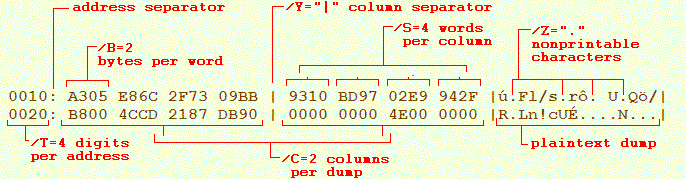# OKdump

produces hexadecimal listing of input file with optional conversion between Czech codepages. Output format is widely configurable.

## Brief description

• 16bit DOS console utility, Intel 386 or better CPU required
• file to file or standard input to output processing
• optional clipping of input file (/F=,/H=)
• configurable output format (/B=, /S=, /C=, /R, /Q)
• skips repeating output lines (/E, /V)
• codepage translation (/A, /D, /I, /K, /L, /M, /W)
• substitutes nonprintable characters (/Z=)
• unlimited file size

## Syntax

Input and output file names are expected as OKDUMP command line parameters. Long file names are not supported. The second file name can be omitted, in this case the hexadecimal dump goes to screen. OKDUMP works like a standard input/output filter when both file names are omitted.

When output file already exists, OKDUMP ask for permission to overwrite: Výstupní soubor už existuje. Přepsat (A/N) ? Press A (=yes) to allow or use parameter /O (=do not ask).

Parameters may start with slash / or hyphen - and are not case sensitive. Some parameters require a value which may be written as in any of the following examples:

` /Header=172813   /h172813   -hdr: 72813   -h0x2A30D    /H2A30Dh`

## Input range

With parameters /H= and /F= the range of processed input can be limited. The former defines starting address (default /H=0), the latter defines ending position (default is entire file size).

## Output format

Default listing format has three areas: the address column, hexadecimal output and plaintext output.

Number of digits in address is set with parameter /T= (default is 8). May be set to decimal with /U or supressed with /@. Address can be biased with constant offset given in parameter /+=. e.g. /+=256 for DOS COM executables.

Hexadecimal listing consists of columns /C=, words /S= and bytes /B=. Columns are optically separated with character defined as /Y= (recomended is /Y="|").

Instead of separate plaintext column on the right we may choose to display text data above its hexadecimal form (/R) or not to display it at all (/Q). Pair of parameters /ASCII, /DOS, /ISO8859-2, /KAM, /LATIN2, /MAC, /WIN1250 allows to convert text between these codepages. Nonprintable characters in plaintext column are replaced with character from parameter /Z= (default is /Z=".").## Built-in formats

Ten useful combinations of formating parameters are prepared using numeric shortcut parameters /1../9.
Use OKUMP /X to show all of them:

```Parameter  output width     replaces
/1          78                /C=2 /S=8 /B=1 /T=8 /Y="|"
/2          66                /C=2 /S=4 /B=2 /T=4 /Y="|"
/3          72             /R /C=1 /S=1 /B=32/T=6 /Y=0
/4          77             /Q /C=1 /S=8 /B=4 /T=4 /Y=0
/5          79             /U /C=4 /S=1 /B=5 /T=5 /Y="!"
/6          78          /@ /R /C=1 /S=1 /B=39
/7         123                /C=2 /S=8 /B=2 /T=5 /Y="|"
/8         142             /R /C=4 /S=1 /B=16/T=6 /Y=0
/9         160                /C=3 /S=1 /B=16/T=5 /Y="|"
/0        unlimited     /@ /Q /C=1 /S=1 /B=unlimited
/=  unformated copy of input which respects /Head= and /Foot=.
```

## Condensed listing

Should the same lines in output listing repeat more than three times, the inner lines will be omitted to spare paper in printer. This behaviour can be supressed with /V.

With /E, on the other hand, we may insert empty lines into listing to get more space for handwritten remarks.

## Alphabetical list of parameters

/A codepage ASCII 7bit
/B= format bytes per word
/C= format columns per dump
/D codepage DOS CP437
/E insert empty lines
/I codepage ISO-8859-2
/K codepage Kamenických
/L codepage PC Latin 2 CP852
/M codepage Macintosh
/N supress title line
/O overwrite output file
/P write Czech manual
/Q suppress text column
/R print text above hexadecimal dump
/S= format words per column
/V show all repeating output lines
/W codepage Windows CP1250
/X list built-in formats
/Y= specify column separator character
/Z= specify surrogate character
`OKDUMP /B=1 /C=2 /H=0 /S=8 /T=8 /Y=0 /Z='.' /+=0`